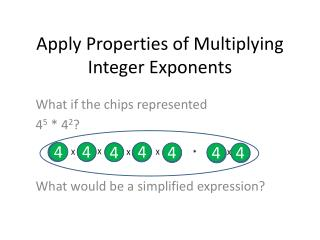DownloadDownload PresentationApply Properties of Multiplying Integer Exponents

# Apply Properties of Multiplying Integer Exponents

Télécharger la présentation## Apply Properties of Multiplying Integer Exponents

- - - - - - - - - - - - - - - - - - - - - - - - - - - E N D - - - - - - - - - - - - - - - - - - - - - - - - - - -
##### Presentation Transcript

1. Apply Properties of Multiplying Integer Exponents What if the chips represented 45 * 42? What would be a simplified expression? 4 4 4 4 4 4 4 X X X X X *

2. Apply Properties of Multiplying Integer Exponents Using your chips, create an equivalent expression for: x4 * x2

3. Apply Properties of Multiplying Integer Exponents What if the circles represented 45 * 4-2?

4. Apply Properties to Multiplying using Integer Exponents What is the new expression? Match positive and negative chips How could you rewrite this expression?

5. Apply Properties to Multiplying using Integer Exponents What is the new expression?

6. Apply Properties of Multiplying Integer Exponents 4 4 4 4 4 X X X X 45* 4-2= 45 * 1/42 = 43 4 4 X

7. Apply Properties of Multiplying Integer Exponents Now use your chips for this problem: x4 * x-2

8. Apply Properties to Dividing using Integer Exponents 4 4 4 4 4 4 X X X X X What if the chips represented 46/ 42? Simplify by reducing to 1 4 4 X

9. Apply Properties to Dividing using Integer Exponents Now you have 1 * What is the simplified value?

10. Apply Properties to Dividing using Integer Exponents 4 4 4 4 4 4 X X X X X What if the circles represented 46/ 4-3? Simplify by reducing to 1 4 4 4

11. Apply Properties to Dividing using Integer Exponents What does 1/4-3 mean? 43

12. Apply Properties to Dividing using Integer Exponents So, 46/ 4-3 really means 46 * 43

13. Apply Properties to Dividing using Integer Exponents What if the circles represented 46/ 46? = 1 Simplify to reduce to 1

14. Apply Properties to Dividing using Integer Exponents What if the circles represented 46/ 46? Simplify to reduce to 1

15. Apply Properties to Dividing using Integer Exponents Only thing left is… 1 So, a zero exponent means….

16. Properties of Integer Exponents Positive exponents Multiplication of integer expressions Negative exponents Zero exponents Division of integer expressions

17. Properties of Integer Exponents Positive exponents Ex: x4 = x * x * x *x 52 = 5 * 5 = 25

18. Properties of Integer Exponents What is a quick way to find the exponent of the result? Multiplying Integer exponents Equivalent to the base multiplied by itself the amount of the exponent x2 * x3 = x? = 2 + 3 = 5

19. Properties of Integer Exponents Negative exponents Equivalent to the reciprocal of base to the positive exponent Ex: x-n = 1/xn 5-2 = (1/5)2 = 1/5 * 1/5 = 1/25 2

20. Properties of Integer Exponents Dividing Integer Exponents Equivalent to the reciprocal of base to the positive exponent X4 / x2 = x? 4 + (-2) = -2 What is a quick way to find the exponent of the result?

21. Properties of Integer Exponents Zero exponents Equivalent to the value 1 Ex: x0 = 1 50 = 1